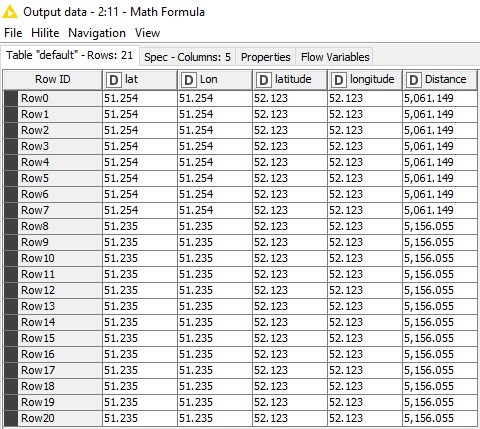# creating new column for distance in Km

Hi all,
I have a question regarding calculation of distance in km I got this table with following data as shown in fig

basically, I have to “Add the Distance in Km” then I have to Label the Distances of foreign countries in table afterward I have to label distance according to categories like the following requirements which are as under:
within 15km from City center => Lokal Customers
From 15 to 50 km from city center => Distant Customer
From 50 km but within Germany => Online shopping Customer
Not in Germany => Foreign Customer
Waiting for quick response. Thank you in advance.
Regards,
Q

So … what’s the question?

Hi,
First you have to use a “Math Formula” or “Column Expressions” node to calculate the distance by using the following formula:

``````dlon = lon2 - lon1
dlat = lat2 - lat1
a = (sin(dlat/2))^2 + cos(lat1) * cos(lat2) * (sin(dlon/2))^2
c = 2 * atan2( sqrt(a), sqrt(1-a) )
distance = R * c (where R is the radius of the Earth - 6,371,000 m)
``````

Then use a “Rule Engine” node to label the output.

Good luck

Thank you I will try it and get back to you. Really thanks alot

I have transformed the formula for you, so that you can use the following directly in a “Math Formula” node:

`6,371 * (2 * atan2( sqrt(((sin((\$latitude\$ - [baseLatitude])/2))^2 + cos([baseLatitude]) * cos(\$latitude\$) * (sin((\$longitude\$ - [base Longitude])/2))^2)), sqrt(1-((sin((\$latitude\$ - [baseLatitude])/2))^2 + cos([baseLatitude]) * cos(\$latitude\$) * (sin((\$longitude\$ - [base Longitude])/2))^2))))`

This formula calculates the distance in km. You have to replace [baseLatitude] and [baseLongitude] with the actual values of your base location. Then the rest of formula will go fine (I hope…).

1 Like

Hi,
What do you mean by base latitiude and longitude means the distance or other because I have four lat / log as shown in figure:

Kindly have look

Sir question is that How to calculate the distance in km and after wards catagorizing accoring to above requirements.
I hope you got it.

Which longitude and latitude are referring to the location of your organization (the location you want to calculate the distance of customers from)? Use them in place of [base…]
and use the other ones (the location of the customers) in place of \$lon…\$

For example if the “latitude” and “longitude” columns are referring to the location of your organization and the “lat” and “Lon” columns are referring to your customers’ location then the formula should be:

`6371 * (2 * atan2( sqrt(((sin((\$longitude\$ - \$Lon\$)/2))^2 + cos(\$latitude\$) * cos(\$lat\$) * (sin((\$latitude\$ - \$lat\$)/2))^2)), sqrt(1-((sin((\$longitude\$ - \$Lon\$)/2))^2 + cos(\$latitude\$) * cos(\$lat\$) * (sin((\$latitude\$ - \$lat\$)/2))^2))))`

Thank you I will try this one and see it. Sir great ful

No not working

Basically one Lat/lon is for city which I got it through shopping platform and other is for country

The distance is obviously already in the last column. It’s 0 (zero) in the screenshot above this post, because both coordinates are equal. So, what exactly is the problem?

`6371 * (2 * atan2( sqrt(((sin((\$longitude\$ - \$Lon\$)/2))^2 + cos(\$latitude\$) * cos(\$lat\$) * (sin((\$latitude\$ - \$lat\$)/2))^2)), sqrt(1-((sin((\$longitude\$ - \$Lon\$)/2))^2 + cos(\$latitude\$) * cos(\$lat\$) * (sin((\$latitude\$ - \$lat\$)/2))^2))))`

I updated the formula. There was a problem in placing the parentheses. (Be aware that I used “latitude” and “longitude” columns as base location and then I used “lat” and “Lon” columns as customers’ location)Now you can use a “Rule Engine” node to label the output.

I’m not sure if I’m getting you clearly but I can say that you need the coordination of the shopping center or anywhere that you consider as base location and the coordination of the customers’ location. Then you can find the distance between these 2 by using the formula I provided in a “Math Formula” node. And after that you can easily use the “Rule Engine” node to put labels on the output data based on the distance you calculated.

Good luck!

Maybe it’s auto generated by the system and he wants to recalculate the distance to make sure the system is working well…!!!1 Like

Thank you for making jokes, anyways as I mentioned before that I want to solve this requirement as under:
within 15km from City centre => Lokal Customers
From 15 to 50 km from city centre => Distant Customer
From 50 km but within Germany => Onlineshopping Customer
Not in Germany => Foreign Customer

Distance I know its there but when I used rule engine the results are not coming. Thank you

Thank you for your help. It works thank you for your effort.